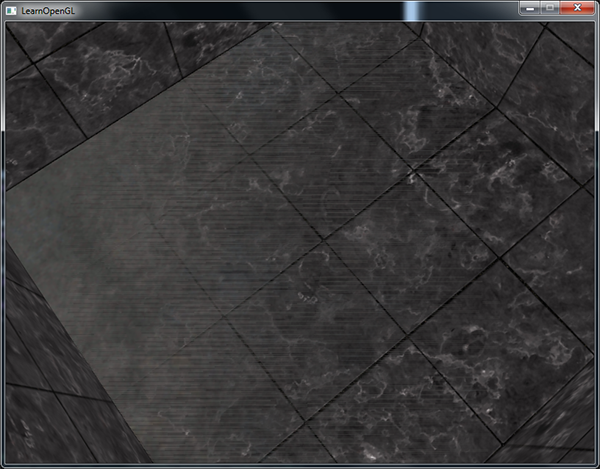# 深度测试

Important

glEnable(GL_DEPTH_TEST);


glClear(GL_COLOR_BUFFER_BIT | GL_DEPTH_BUFFER_BIT);


glDepthMask(GL_FALSE);


## 深度测试函数

OpenGL允许我们修改深度测试中使用的比较运算符。这允许我们来控制OpenGL什么时候该通过或丢弃一个片段，什么时候去更新深度缓冲。我们可以调用glDepthFunc函数来设置比较运算符（或者说深度函数(Depth Function)）：

glDepthFunc(GL_LESS);


GL_ALWAYS 永远通过深度测试
GL_NEVER 永远不通过深度测试
GL_LESS 在片段深度值小于缓冲的深度值时通过测试
GL_EQUAL 在片段深度值等于缓冲区的深度值时通过测试
GL_LEQUAL 在片段深度值小于等于缓冲区的深度值时通过测试
GL_GREATER 在片段深度值大于缓冲区的深度值时通过测试
GL_NOTEQUAL 在片段深度值不等于缓冲区的深度值时通过测试
GL_GEQUAL 在片段深度值大于等于缓冲区的深度值时通过测试

glEnable(GL_DEPTH_TEST);
glDepthFunc(GL_ALWAYS);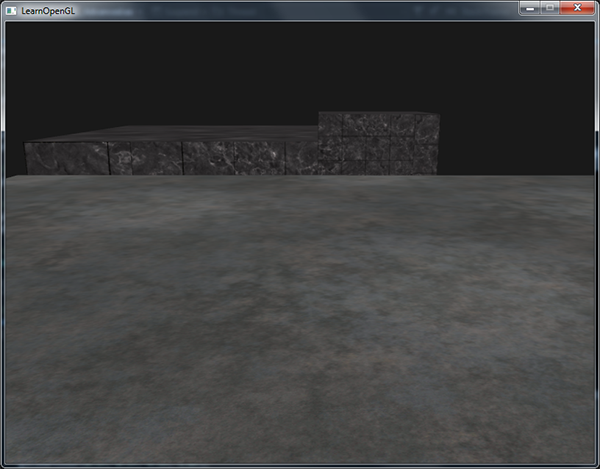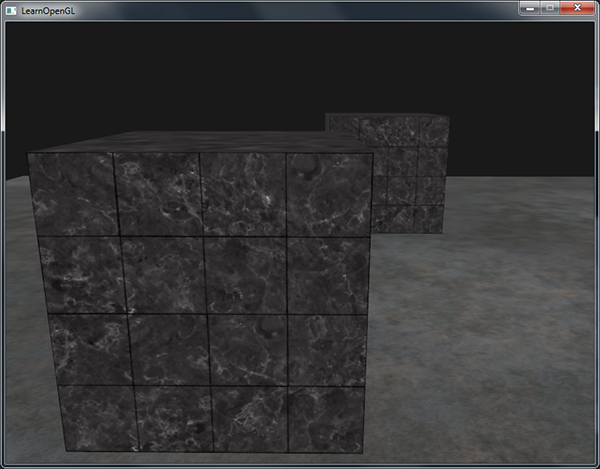## 深度值精度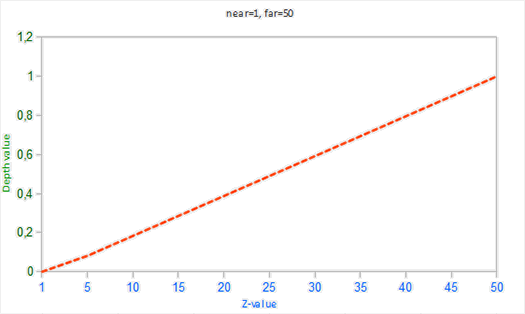Important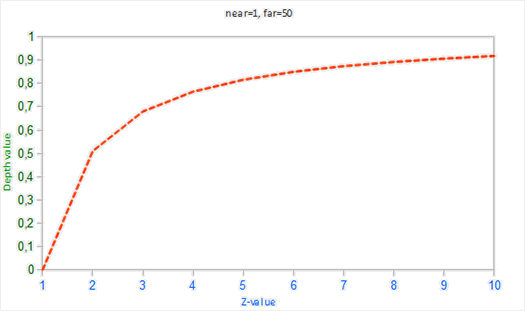## 深度缓冲的可视化

void main()
{
FragColor = vec4(vec3(gl_FragCoord.z), 1.0);
}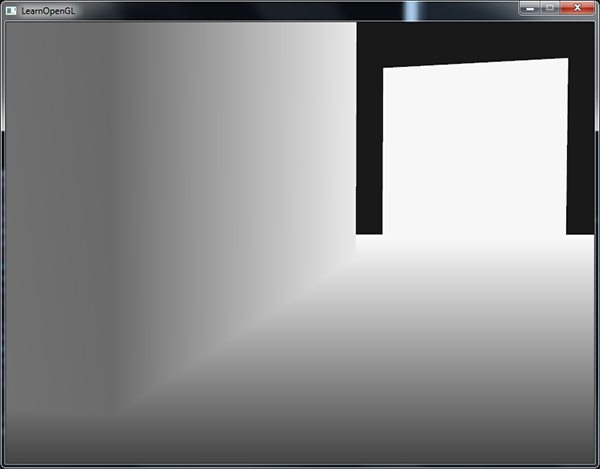float z = depth * 2.0 - 1.0;


float linearDepth = (2.0 * near * far) / (far + near - z * (far - near));


#version 330 core
out vec4 FragColor;

float near = 0.1;
float far  = 100.0;

float LinearizeDepth(float depth)
{
float z = depth * 2.0 - 1.0; // back to NDC
return (2.0 * near * far) / (far + near - z * (far - near));
}

void main()
{
float depth = LinearizeDepth(gl_FragCoord.z) / far; // 为了演示除以 far
FragColor = vec4(vec3(depth), 1.0);
}## 深度冲突